This book is archived and will be removed July 6, 2022. Please use the updated version.

17 Sound

# 17.5 Sources of Musical Sound

### Learning Objectives

By the end of this section, you will be able to:

• Describe the resonant frequencies in instruments that can be modeled as a tube with symmetrical boundary conditions
• Describe the resonant frequencies in instruments that can be modeled as a tube with anti-symmetrical boundary conditions

Some musical instruments, such as woodwinds, brass, and pipe organs, can be modeled as tubes with symmetrical boundary conditions, that is, either open at both ends or closed at both ends ((Figure)). Other instruments can be modeled as tubes with anti-symmetrical boundary conditions, such as a tube with one end open and the other end closed ((Figure)).

Resonant frequencies are produced by longitudinal waves that travel down the tubes and interfere with the reflected waves traveling in the opposite direction. A pipe organ is manufactured with various tubes of fixed lengths to produce different frequencies. The waves are the result of compressed air allowed to expand in the tubes. Even in open tubes, some reflection occurs due to the constraints of the sides of the tubes and the atmospheric pressure outside the open tube.

The antinodes do not occur at the opening of the tube, but rather depend on the radius of the tube. The waves do not fully expand until they are outside the open end of a tube, and for a thin-walled tube, an end correction should be added. This end correction is approximately 0.6 times the radius of the tube and should be added to the length of the tube.

Players of instruments such as the flute or oboe vary the length of the tube by opening and closing finger holes. On a trombone, you change the tube length by using a sliding tube. Bugles have a fixed length and can produce only a limited range of frequencies.

The fundamental and overtones can be present simultaneously in a variety of combinations. For example, middle C on a trumpet sounds distinctively different from middle C on a clarinet, although both instruments are modified versions of a tube closed at one end. The fundamental frequency is the same (and usually the most intense), but the overtones and their mix of intensities are different and subject to shading by the musician. This mix is what gives various musical instruments (and human voices) their distinctive characteristics, whether they have air columns, strings, sounding boxes, or drumheads. In fact, much of our speech is determined by shaping the cavity formed by the throat and mouth, and positioning the tongue to adjust the fundamental and combination of overtones. For example, simple resonant cavities can be made to resonate with the sound of the vowels ((Figure)). In boys at puberty, the larynx grows and the shape of the resonant cavity changes, giving rise to the difference in predominant frequencies in speech between men and women.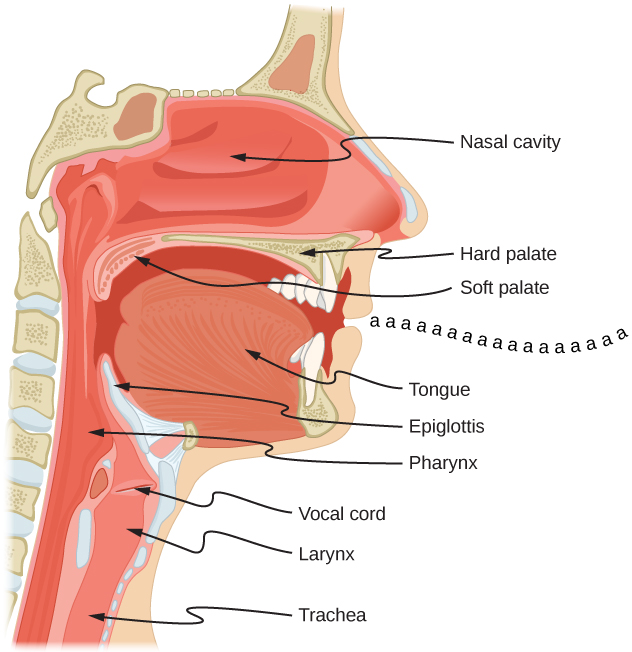Figure 17.26 The throat and mouth form an air column closed at one end that resonates in response to vibrations in the voice box. The spectrum of overtones and their intensities vary with mouth shaping and tongue position to form different sounds. The voice box can be replaced with a mechanical vibrator, and understandable speech is still possible. Variations in basic shapes make different voices recognizable.

### Example

#### Finding the Length of a Tube with a 128-Hz Fundamental

(a) What length should a tube closed at one end have on a day when the air temperature is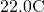if its fundamental frequency is to be 128 Hz (C below middle C)?

(b) What is the frequency of its fourth overtone?

#### Strategy

The length L can be found from the relationship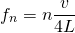, but we first need to find the speed of sound v.

#### Solution

1. Identify knowns: The fundamental frequency is 128 Hz, and the air temperature is.Useto find the fundamental frequency (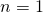),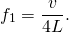Solve this equation for length,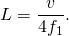Find the speed of sound using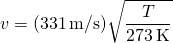,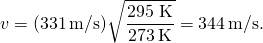Enter the values of the speed of sound and frequency into the expression for L.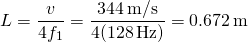2. Identify knowns: The first overtone has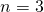, the second overtone has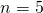, the third overtone has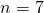, and the fourth overtone has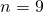.Enter the value for the fourth overtone into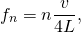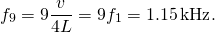#### Significance

Many wind instruments are modified tubes that have finger holes, valves, and other devices for changing the length of the resonating air column and hence, the frequency of the note played. Horns producing very low frequencies require tubes so long that they are coiled into loops. An example is the tuba. Whether an overtone occurs in a simple tube or a musical instrument depends on how it is stimulated to vibrate and the details of its shape. The trombone, for example, does not produce its fundamental frequency and only makes overtones.

If you have two tubes with the same fundamental frequency, but one is open at both ends and the other is closed at one end, they would sound different when played because they have different overtones. Middle C, for example, would sound richer played on an open tube, because it has even multiples of the fundamental as well as odd. A closed tube has only odd multiples.

### Resonance

Resonance occurs in many different systems, including strings, air columns, and atoms. As we discussed in earlier chapters, resonance is the driven or forced oscillation of a system at its natural frequency. At resonance, energy is transferred rapidly to the oscillating system, and the amplitude of its oscillations grows until the system can no longer be described by Hooke’s law. An example of this is the distorted sound intentionally produced in certain types of rock music.

Wind instruments use resonance in air columns to amplify tones made by lips or vibrating reeds. Other instruments also use air resonance in clever ways to amplify sound. (Figure) shows a violin and a guitar, both of which have sounding boxes but with different shapes, resulting in different overtone structures. The vibrating string creates a sound that resonates in the sounding box, greatly amplifying the sound and creating overtones that give the instrument its characteristic timbre. The more complex the shape of the sounding box, the greater its ability to resonate over a wide range of frequencies. The marimba, like the one shown in (Figure), uses pots or gourds below the wooden slats to amplify their tones. The resonance of the pot can be adjusted by adding water.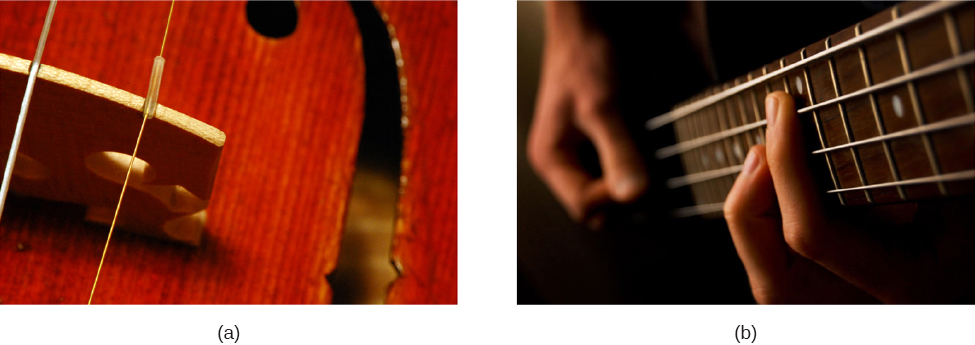Figure 17.27 String instruments such as (a) violins and (b) guitars use resonance in their sounding boxes to amplify and enrich the sound created by their vibrating strings. The bridge and supports couple the string vibrations to the sounding boxes and air within. (credit a: modification of work by Feliciano Guimares; credit b: modification of work by Steve Snodgrass)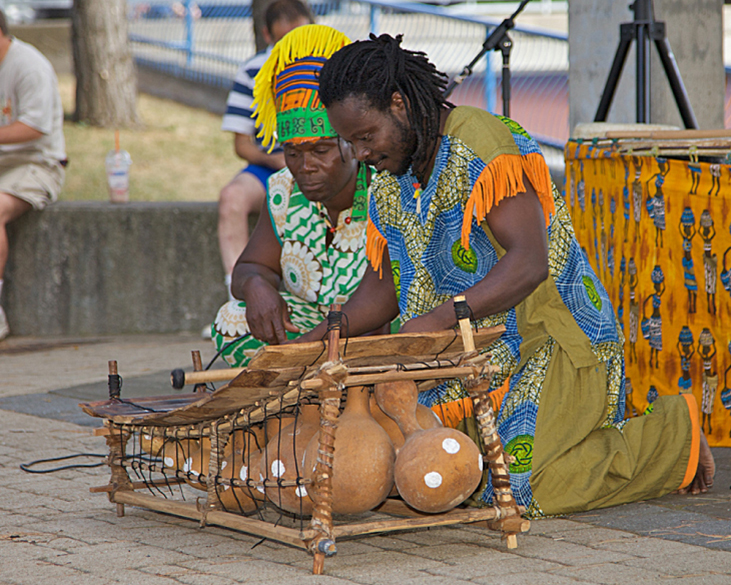Figure 17.28 Resonance has been used in musical instruments since prehistoric times. This marimba uses gourds as resonance chambers to amplify its sound. (credit: “APC Events”/Flickr)

We have emphasized sound applications in our discussions of resonance and standing waves, but these ideas apply to any system that has wave characteristics. Vibrating strings, for example, are actually resonating and have fundamentals and overtones similar to those for air columns. More subtle are the resonances in atoms due to the wave character of their electrons. Their orbitals can be viewed as standing waves, which have a fundamental (ground state) and overtones (excited states). It is fascinating that wave characteristics apply to such a wide range of physical systems.

### Summary

• Some musical instruments can be modeled as pipes that have symmetrical boundary conditions: open at both ends or closed at both ends. Other musical instruments can be modeled as pipes that have anti-symmetrical boundary conditions: closed at one end and open at the other.
• Some instruments, such as the pipe organ, have several tubes with different lengths. Instruments such as the flute vary the length of the tube by closing the holes along the tube. The trombone varies the length of the tube using a sliding bar.
• String instruments produce sound using a vibrating string with nodes at each end. The air around the string oscillates at the frequency of the string. The relationship for the frequencies for the string is the same as for the symmetrical boundary conditions of the pipe, with the length of the pipe replaced by the length of the string and the velocity replaced by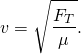### Conceptual Questions

How does an unamplified guitar produce sounds so much more intense than those of a plucked string held taut by a simple stick?

Consider three pipes of the same length (L). Pipe A is open at both ends, pipe B is closed at both ends, and pipe C has one open end and one closed end. If the velocity of sound is the same in each of the three tubes, in which of the tubes could the lowest fundamental frequency be produced? In which of the tubes could the highest fundamental frequency be produced?

When resonating at the fundamental frequency, the wavelength for pipe C is 4L, and for pipes A and B is 2L. The frequency is equal to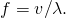Pipe C has the lowest frequency and pipes A and B have equal frequencies, higher than the one in pipe C.

Pipe A has a length L and is open at both ends. Pipe B has a length L/2 and has one open end and one closed end. Assume the speed of sound to be the same in both tubes. Which of the harmonics in each tube would be equal?

A string is tied between two lab posts a distance L apart. The tension in the string and the linear mass density is such that the speed of a wave on the string is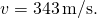A tube with symmetric boundary conditions has a length L and the speed of sound in the tube isWhat could be said about the frequencies of the harmonics in the string and the tube? What if the velocity in the string were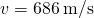?

Since the boundary conditions are both symmetric, the frequencies are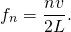Since the speed is the same in each, the frequencies are the same. If the wave speed were doubled in the string, the frequencies in the string would be twice the frequencies in the tube.

### Problems

If a wind instrument, such as a tuba, has a fundamental frequency of 32.0 Hz, what are its first three overtones? It is closed at one end. (The overtones of a real tuba are more complex than this example, because it is a tapered tube.)

What are the first three overtones of a bassoon that has a fundamental frequency of 90.0 Hz? It is open at both ends. (The overtones of a real bassoon are more complex than this example, because its double reed makes it act more like a tube closed at one end.)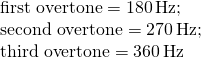How long must a flute be in order to have a fundamental frequency of 262 Hz (this frequency corresponds to middle C on the evenly tempered chromatic scale) on a day when air temperature is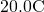? It is open at both ends.

What length should an oboe have to produce a fundamental frequency of 110 Hz on a day when the speed of sound is 343 m/s? It is open at both ends.

(a) Find the length of an organ pipe closed at one end that produces a fundamental frequency of 256 Hz when air temperature is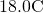. (b) What is its fundamental frequency at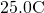?

An organ pipe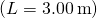is closed at both ends. Compute the wavelengths and frequencies of the first three modes of resonance. Assume the speed of sound is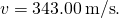The pipe has symmetrical boundary conditions;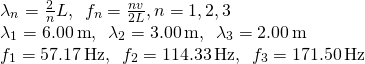An organ pipeis closed at one end. Compute the wavelengths and frequencies of the first three modes of resonance. Assume the speed of sound isA sound wave of a frequency of 2.00 kHz is produced by a string oscillating in the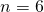mode. The linear mass density of the string is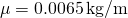and the length of the string is 1.50 m. What is the tension in the string?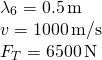Consider the sound created by resonating the tube shown below. The air temperature is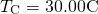. What are the wavelength, wave speed, and frequency of the sound produced?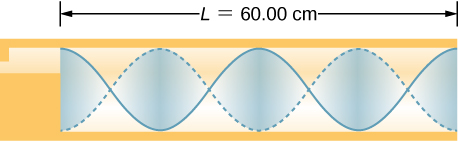A student holds an 80.00-cm lab pole one quarter of the length from the end of the pole. The lab pole is made of aluminum. The student strikes the lab pole with a hammer. The pole resonates at the lowest possible frequency. What is that frequency?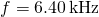A string on the violin has a length of 24.00 cm and a mass of 0.860 g. The fundamental frequency of the string is 1.00 kHz. (a) What is the speed of the wave on the string? (b) What is the tension in the string?

By what fraction will the frequencies produced by a wind instrument change when air temperature goes from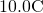to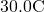? That is, find the ratio of the frequencies at those temperatures.

*** QuickLaTeX cannot compile formula:
$3\text{%}$

*** Error message:
File ended while scanning use of \text@.
Emergency stop.